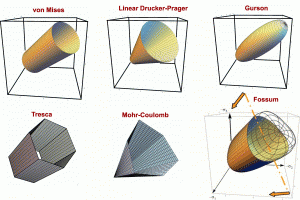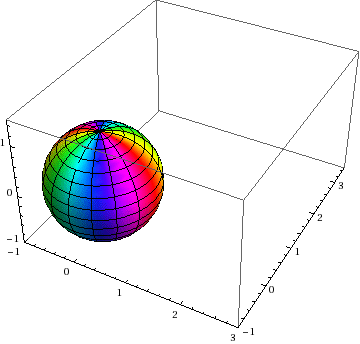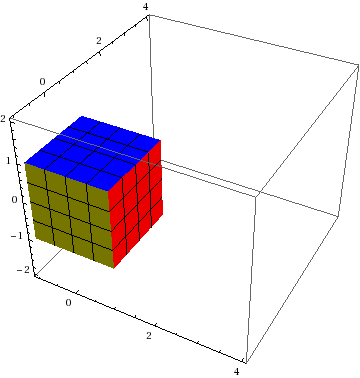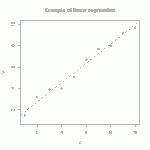# Aldridge (AKA Blake) spherical source verification test for dynamic continuum codes

This post has the following aims:

• Provide documentation and source code for a spherically symmetric wave propagation in a linear-elastic medium.
• Tell a story illustrating how this simple verification problem helped to validate a complicated rate-dependent and history-dependent geomechanics model.
• Warn against believing previously reported material parameters, since they might have been the result of constitutive parameter tweaking to compensate for unrelated errors in the host code. Continue reading

# Publication: A model for statistical variation of fracture properties in a continuum mechanics code

NEWS FLASH: The print version of the Meyer-Brannon paper on statistical variation of fracture patterns in a continuum code (CTH) is now available at http://dx.doi.org/10.1016/j.ijimpeng.2010.09.007.

# Some topics in rock and brittle media modeling

The following slides are taken primarily from a standard collection that has been used over the last several years to introduce mechanics researchers to concepts such as third-invariant dependence of material failure, softening, mesh dependency, the need for regularization through introduction of a length scale, Weibull statistics in strength data, etc.

# Publication: Elements of Phenomenological Plasticity: Geometrical Insight, Computational Algorithms, and Topics in Shock PhysicsThis 2007 Book Chapter on the basics of plasticity theory reviews the terminology and governing equations of plasticity, with emphasis on amending misconceptions, providing physical insights, and outlining computational algorithms. Plasticity theory is part of a larger class of material models in which a pronounced change in material response occurs when the stress (or strain) reaches a critical threshold level. If the stress state is subcritical, then the material is modeled by classical elasticity. The bound- ary of the subcritical (elastic) stress states is called the yield surface. Plasticity equations apply if continuing to apply elasticity theory would predict stress states that extend beyond this the yield surface. The onset of plasticity is typically characterized by a pronounced slope change in a stress–strain dia-gram, but load reversals in experiments are necessary to verify that the slope change is not merely nonlinear elasticity or reversible phase transformation.
The threshold yield surface can appear to be significantly affected by the loading rate, which has a dominant effect in shock physics applications.

In addition to providing a much-needed tutorial survey of the governing equations and their solution (defining Lode angle and other Lode invariants and addressing the surprisingly persistent myth that closest-point return satisfies the governing equations), this book chapter includes some distinctive contributions such as a simple 2d analog of plasticity that exhibits the same basic features of plasticity (such as existence of a “yield” surface with associative flow and vertex theory), an extended discussion of apparent nonassociativity, stability and uniqueness concerns about nonassociativity, and a  summary of apparent plastic wave speeds in relation to elastic wave speeds (especially noting that non-associativity admits plastic waves that travel faster than elastic waves).

For the full manuscript with errata, click 2007 Book Chapter on the basics of plasticity theory.

# Streamline visualization of tensor fields in solid mechanicsBrazilian stress net before and after material failure. Colors indicate maximum principal stress (showing tension in the center of this axially compressed disk). Lines show directions of max principal stress.

A stress net is simply a graphical depiction of principal stress directions (or other directions derived from them, such as rotating them by 45 degrees to get the maximum shear lines.)  Continue reading

# Annulus Twist as a verification test

Illustrated below is the solution to an idealized problem of a linear elastic annulus (blue) subjected to twisting motion caused by rotating the T-bar an angle$\alpha$.  The motion is presumed to be applied slowly enough that equilibrium is satisfied.This simple problem is taken to be governed by the equations of equilibrium$\vec{\nabla}\cdot\sigma=0$, along with the plane strain version of Hooke’s law in which Cauchy stress is taken to be linear with respect to the small strain tensor (symmetric part of the displacement gradient).  If this system of governing equations is implemented in a code, the code will give you an answer, but it is up to you to decide if that answer is a reasonable approximation to reality. This observation helps to illustrate the distinction between verification (i.e., evidence that the equations are solved correctly) and validation (evidence that physically applicable and physically appropriate equations are being solved).  The governing equations always have a correct answer (verification), but that answer might not be very predictive of reality (validation).

# Tutorial: Visualizing Deformation

If you’ve never heard of a continuum mapping, read our introduction to mappings.

This posting discusses the two most common visualization methods for 3D homogeneous mappings: Showing how a sphere transforms to an ellipsoid and how a cube transforms to a parallelepiped:# Resource: Perfect triples, “nice” unit vectors, and “nice” orthogonal matrices

“NICE” lists:

Have you ever noticed that textbooks often involve so-called 3-4-5 triangles? They do that to make the algebraic manipulations easier for students.  If the two legs of a right triangle are of length 3 and 4, then the hypotenuse (found from the Pythagorean theorem) has a length of 5, which is “nice” in the sense that it is an integer rather than an irrational square root that more typically comes from solving the Pythagorean theorem. As discussed in many elementary math sites (such as MakingMathematics.org), another example of a “nice” triangle is the 5-12-13 triangle, since$5^2+12^2=13^2$.

The external links in this posting contain a list of more of these so-called perfect triples of integers$\{a,b,c\}$ for which$a^2+b^2=c^2$. Perfect triples are also used to create “nice” 2D unit vectors whose components are each rational numbers (instead of involving irrational square roots from the normalization process). For example, the classic unit vector based on the 3-4-5 perfect triple is simply$\{\frac{3}{5},\frac{4}{5}\}$. Continue reading

# Tutorial: multi-linear regressionThe straight line is the linear regression of a function that takes scalars (x-values) as input and returns scalars (y-values) as output. (figure from GANFYD)

You’ve probably seen classical equations for linear regression, which is a procedure that finds the straight line that best fits a set of discrete points$\{(x_1,y_1), (x_2,y_2),...,(x_N,y_N)\}$. You might also be aware that similar formulas exist to find a straight line that is a best (least squares) fit to a continuous function$y(x)$.The pink parallelogram is the multi-linear regression of a function that takes vectors (gray dots) as input and returns vectors (blue dots) as output

The bottom of this post provides a link to a tutorial on how to generalize the concept of linear regression to fit a function$\vec{y}(\vec{x})$ that takes a vector$\vec{x}$ as input and produces a vector$\vec{y}$ as output. In mechanics, the most common example of this type of function is a mapping function that describes material deformation: the input vector is the initial location of a point on a body, and the output vector is the deformed location of the same point. The image shows a collection of input vectors (initial positions, as grey dots) and a collection of output vectors (deformed locations as blue dots). The affine fit to these descrete data is the pink parallelogram. Continue reading

# Publication: A model for statistical variation of fracture properties in a continuum mechanics code

H.W. Meyer Jr. and R.M. Brannon

[This post refers to the original on-line version of the publication. The final (paper) version with page numbers and volume is found at http://dx.doi.org/10.1016/j.ijimpeng.2010.09.007. Some further details and clarifications are in the 2012 posting about this article]

Continuum mechanics codes modeling failure of materials historically have considered those materials to be homogeneous, with all elements of a material in the computation having the same failure properties. This is, of course, unrealistic but expedient. But as computer hardware and software has evolved, the time has come to investigate a higher level of complexity in the modeling of failure. The Johnsone-Cook fracture model is widely used in such codes, so it was chosen as the basis for the current work. The CTH ﬁnite difference code is widely used to model ballistic impact and penetration, so it also was chosen for the current work. The model proposed here does not consider individual ﬂaws in a material, but rather varies a material’s Johnsone-Cook parameters from element to element to achieve in homogeneity. A Weibull distribution of these parameters is imposed, in such a way as to include a size effect factor in the distribution function. The well-known size effect on the failure of materials must be physically represented in any statistical failure model not only for the representations of bodies in the simulation (e.g., an armor plate), but also for the computational elements, to mitigate element resolution sensitivity of the computations.The statistical failure model was tested in simulations of a Behind Armor Debris (BAD) experiment, and found to do a much better job at predicting the size distribution of fragments than the conventional (homogeneous) failure model. The approach used here to include a size effect in the model proved to be insufﬁcient, and including correlated statistics and/or ﬂaw interactions may improve the model.

Available Online:

http://www.mech.utah.edu/~brannon/pubs/7-2011MeyerBrannon_IE_1915_final_onlinePublishedVersion.pdf

http://www.sciencedirect.com/science/article/pii/S0734743X10001466